# Rayleigh distribution

(diff) ← Older revision | Latest revision (diff) | Newer revision → (diff)

A continuous probability distribution with densitydepending on a scale parameter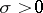. A Rayleigh distribution has positive asymmetry; its unique mode is at the point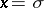. All moments of a Rayleigh distribution are finite, the mathematical expectation and variance being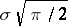and, respectively. The distribution function of a Rayleigh distribution has the form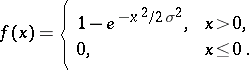A Rayleigh distribution is a special case of the distribution with density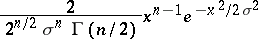when; hence, whenthe Rayleigh distribution coincides with the distribution of the square root of a random variable which has the "chi-squared" distribution with two degrees of freedom. In other words, a Rayleigh distribution can be interpreted as the distribution of the length of a vector in a plane Cartesian coordinate system, the coordinates of which are independent and have the normal distribution with parameters 0 and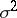. In the three-dimensional space the Maxwell distribution plays a role analogous to the Rayleigh distribution.

A Rayleigh distribution is mainly applied in target theory and statistical communication theory. It was first considered by Lord Rayleigh in 1880 as the distribution of the amplitude resulting from the addition of harmonic oscillations.